© | Dror Bar-Natan: Classes: 2009-10: AKT:

# Video AKT-090917-1

 width: 320 720 download ogg/AKT-090917-1_320.ogg For now, this video can only be viewed with web browsers that support

Videography by Karene Chu

Notes on AKT-090917-1:    [edit, refresh]

The definition of finite type, weight systems, Jones is a finite type series.

# Week of... Videos, Notes, and Links
: 3-colourings, Reidemeister's theorem, invariance, the Kauffman bracket.
: R23 invariance of the bracket, R1, the writhe, the Jones polynomial, programming the Jones polynomial.
Tricolourability
2 Sep 14 : More on Jones, some pathologies and more on Reidemeister, our overall agenda.
: The definition of finite type, weight systems, Jones is a finite type series.
: The skein relation for Jones; HOMFLY-PT and Conway; the weight system of Jones.
3 Sep 21 : FI, 4T, HOMFLY and FI and 4T, statement of the Fundamental Theorem, framed knots.
: Some dimensions of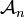${\mathcal A}_n$,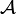${\mathcal A}$ is a commutative algebra,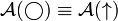${\mathcal A}(\bigcirc)\equiv{\mathcal A}(\uparrow)$.
Class Photo
:${\mathcal A}$ is a co-commutative algebra, the relation with products of invariants,${\mathcal A}$ is a bi-algebra.
4 Sep 28 Homework Assignment 1
Homework Assignment 1 Solutions
: The Milnor-Moore theorem, primitives, the map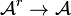${\mathcal A}^r\to{\mathcal A}$.
: Jacobi diagrams, AS, IHX, STU, and the equivalence of all that with 4T.
: The very basics on Lie algebras.
5 Oct 5 : Lie algebraic weight systems,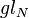$gl_N$.
: More on$gl_N$, Lie algebras and the four colour theorem.
: The "abstract tenssor" approach to weight systems,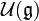${\mathcal U}({\mathfrak g})$ and PBW, the map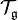${\mathcal T}_{\mathfrak g}$.
6 Oct 12 : Algebraic properties of${\mathcal U}({\mathfrak g})$ vs. algebraic properties of${\mathcal A}$.
Thursday's class canceled.
7 Oct 19 : Universal finite type invariants, filtered and graded spaces, expansions.
Homework Assignment 2
The Stonehenge Story
: The Stonehenge Story to IHX and STU.
: The Stonhenge Story: anomalies, framings, relation with physics.
8 Oct 26 : Knotted trivalent graphs and their chord diagrams.
: Zsuzsi Dancso on the Kontsevich Integral (1).
: Zsuzsi Dancso on the Kontsevich Integral (2).
9 Nov 2 : The details of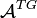${\mathcal A}^{TG}$.
: Three basic problems: genus, unknotting numbers, ribbon knots.
: The three basic problems and algebraic knot theory.
10 Nov 9 : Tangles and planar algebras, shielding and the generators of KTG.
Homework Assignment 3
No Thursday class.
11 Nov 16 Local Khovanov Homology
: Local Khovanov homology, I.
: Local Khovanov homology, II.
12 Nov 23 : Emulation of one structure inside another, deriving the pentagon.
: Peter Lee on braided monoidal categories, I.
: Peter Lee on braided monoidal categories, II.
13 Nov 30 : The relations in KTG.
: The Existence of the Exponential Function.
: The Final Exam, Dror's failures.
F Dec 7 The Final Exam on Thu Dec 10, 9-11, Bahen 6183.
Register of Good Deeds / To Do List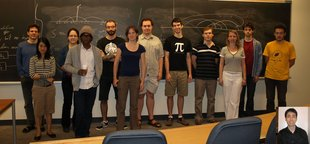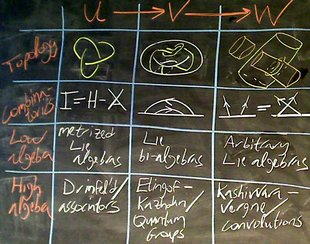refresh
panel
Managed by dbnvp: Drag red strip to resize.0:00:00  Today and for a little further we will be following my 1992 paper, On the Vassiliev Knot Invariants.0:01:03  Computing the tricolouring invariant efficiently: A priori, it is an exponential time problem since for a knot diagram with$n$ arcs we need to consider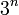$3^n$ possible colourings. However, the problem can be reduced to Gaussian elimination over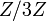$Z/3Z$ and quadratic time. For more details see AKT-09/Tricolourability.0:05:25  Correction on last time's correction on Jones polynomial: Instead of substituting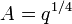$A=q^{1/4}$, we should substitute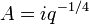$A= i q^{-1/4}$ (For knots, this is same as setting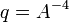$q = A^{-4}$ since$A$ only appear in terms of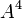$A^4$)0:08:22  Universal polynomial invariant: Looking at polynomials defined on the space of knots0:10:01  What is a polynomial: Definition for both continuous and discrete spaces (higher derivatives or differences vanish)0:11:09  Defining 'derivative' for maps from the space of oriented$n$-singular knots embedded in an oriented copy of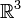$\mathbb{R}^3$ to Abelian group$A$. (Given knot invariant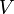$V$,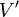$V'$ maps$n$-singular knots to sums of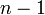$n-1$-singular knots and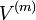$V^{(m)}$ maps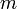$m$-singular knots to$A$ )0:11:19  Let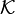$\mathcal{K}$ denote the space of oriented knots in an oriented$\mathbb{R}^3$ and$A$ be any abelian group. Then, given any invariant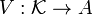$V: \mathcal{K} \rightarrow A$, we can extend$V$ to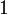$1$-singular knots (i.e. knots with one double point) by setting:

Failed to parse (unknown function\doublepoint): V^{(1)}(\doublepoint)=V(\overcrossing) - V(\undercrossing)

This is analogous to taking the first derivative.0:15:48  Likewise, for an$m$-singular knot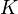$K$, we can define: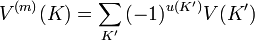$V^{(m)}(K)=\sum_{K'}{(-1)^{u(K')}V(K')}$

where the sum is over the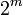$2^m$ resolutions of$K$ and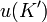$u(K')$ is the number of under resolutions made in obtaining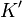$K'$.

Parallel to the result in calculus that partial derivatives commute, we have that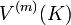$V^{(m)}(K)$ is independent of the order in which the double points are resolved.0:20:08  Definition: A knot invariant$V$ is of Vassiliev type$m$ if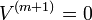$V^{(m+1)} = 0$ (on the whole space of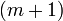$(m+1)$-singular knots).

Notation: We drop the superscript in$V^{(m)}$ since for each$m$,$V^{(m)}$ is only defined for$m$-singular knots.

We can also express the 'type$m$' condition as:

Failed to parse (unknown function\doublepoint): V(\doublepoint ... \doublepoint)=0

whenever we have more than$m$ double points.0:22:10  Motivation: instead of studying the knot invariant itself, we may study the derivatives of it.0:23:37  Let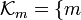$\mathcal{K}_m = \{ m$-singular knots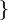$\}$

Given$V$ of type$m$, We have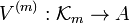$V^{(m)}: \mathcal{K}_m \rightarrow A$.

Since$V^{(m+1)}=0$,$V^{(m)}$ does not distinguish over crossing and under crossings in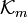$\mathcal{K}_m$.

Let Failed to parse (unknown function\overcrossing): \mathcal{D}_m = \mathcal{K}_m / (\overcrossing=\undercrossing) .

Hence, the weight system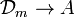$\mathcal{D}_m \rightarrow A$ given by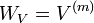$W_V = V^{(m)}$ is well-defined.0:28:15  Claim: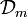$\mathcal{D}_m$ corresponds to the set of chord diagrams. (Deduce that there are at most finitely many linearly independent invariants of type$m$.)0:31:51  Proof of the claim: Walking along the knot and mark the singular points.0:46:11  Let$K$ be a knot,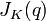$J_K(q)$ be its Jones polynomial. Substitute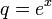$q = e^x$ and expand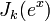$J_k(e^x)$ into power series. We have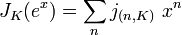$J_K(e^x) = \sum_n j_{(n,K)} \ x^n$ where the coefficients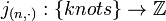$j_{(n,\cdot)}: \{knots \} \rightarrow \mathbb{Z}$ are knot invariants.

Thm: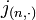$j_{(n, \cdot)}$ is of type$n$.